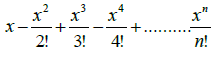# CBSE Class 11 Computer Science Question Paper Set O Solved

Read and download CBSE Class 11 Computer Science Question Paper Set O Solved designed as per the latest question paper pattern and Class 11 examination guidelines issued by CBSE, NCERT and KVS. The past year Question Papers for Class 11 Computer Science have been provided with solutions which will help students to assess their performance and find out topics in Computer Science grade 11 which they need to improve to get better marks in Standard 11 exams. After solving these last year papers also refer to solved Sample Papers for Class 11 Computer Science available on our website to build strong understanding of the subject

## Computer Science Question Paper Class 11

Students can refer to the below Class 11 Computer Science Question Paper designed to help students understand the pattern of questions that will be asked in Grade 11 exams. Please download CBSE Class 11 Computer Science Question Paper Set O Solved

### Computer Science Class 11 Question Paper

1. a. What is the difference between RAM and ROM?

b. Explain Parallel Port.

c. What is the difference between CISC and RISC Computers?

d. What do you mean by Bluetooth? Explain.

e. What is a Cache memory? Why it is considered crucial for a microprocessors performance?

f. What are the basic characteristics of a Microprocessor?

g. Explain :
i) InfraRed Port       ii) PS-2 Port

h. Write a note on:
i) DVD’s                ii) Hard Disks

2. a. What will be the sizes of following constants?
„\a‟   and   “\a”

b. Differentiate between a Syntax error and Logical error with examples.

c. Explain the following terms with examples:
i) Keywords        ii) Escape sequence

3. a. What is a reference variable? What is its usage?

b. Give the output of the following code:
#include<iostream.h>
#include<iomanip.h>
void main( )
{ cout<< setw(7) << 6666 <<endl;
cout.setf(ios::fixed);
cout<< setprecision(4) << 36.42187;
}

c. What is the difference between fundamental and derived data type explain with an example?

4. a. What do you mean by type casting? Explain with an example.

b. Use conditional operator, to represent the following in C++ :
If income>20000, tax=3000 otherwise tax=1500.

c. Given the following expressions:

(a) Avg = 70 (b) Avg == 70
i) How are these two different?
ii) What will be the result of (a) and (b) if the value of Avg is 80 initially?

d. Construct logical expressions to represent the following conditions:
i) z is odd and negative
ii) donation is in the range 3000 - 4000 or guest is 2

e. Write the output of the following:
i) int x, y, z=4;
y=(x=2, x+z);
cout<< z * y << ‟\t‟ <<y;
ii) int m=5;
int w = ++m + 50;
int y = m-- + 40;
cout<<“w= ”<<w<<”\n”<< “y= ”<<y;

f. Write the corresponding C++ expressions for the following mathematical expressions:5. a. Write one limitation and one advantage of a switch statement.

b. Briefly explain Infinite loop with suitable example.

c. What is the role of break and continue statement in loop?

d. Rewrite the following code using while loop:

for(int x=5; x<20; x+=2)
cout<< x << endl;
cout<< “end of loop”;

e. Predict the output of the following C++ program:

#include<iostream.h>
void main()
{ for(int outer=0 ;outer<2; outer++)
{ for(int inner=1; inner<=7; inner=inner+2)
cout<< inner<<“ “;
cout<< endl; } }

f. Rewrite the following program after removing the syntactical error(s), if any. Underline each correction.

#include<iostream.h>
void main( )
{int num=4;
do
{ ans=*num;
cout<ans;
} while(num<10) }

g. Rewrite the following code using switch-case statement:

void main( )
{char ch;
cin >> ch;
if(ch == „R‟)
cout<<“Colour is Red ”;
else if(ch == „W‟)
cout<<“ Colour is White ”;
else
cout<<“Colour is other than Red and White ”;
}

6. a. Write a program to input three integers and print the smallest of the three.

b. Write a program to accept the number of terms and print the Fibonacci series.
0 1 1 2 3 5 8 13 ………

c. Write a C++ program to generate the following pattern:
A
A B
A B C
A B C D

d. Write a program to accept two integers and display the LCM and GCD of the two integers. e.g. If the numbers are 4 and 6 then, LCM = 12 and GCD = 2.

7. a. Write a program to accept Sales from the user. Calculate and display commission for the salesman according to the following rates:

Sales                      Commission Rate

30001 onwards         15% of Sales
22001 - 30000         10% of Sales
12001 – 22000          7 % of Sales
5001 – 12000            3% of Sales
0 - 5000                    0% of Sales

1. Factorial of a number
2. To print ASCII code of an uppercase alphabet.
3. Quit

The program reads a choice (1,2 or 3) from the user and perform the following:
If choice is 1, read an integer and print its factorial.(eg. If number is 5 , output is 120).
If choice is 2, reads an uppercase alphabet and print its equivalent ASCII code. (eg. If
alphabet is B then code is 66).
If choice is 3, then the program should terminate.
Any other choice should display an error message.
The menu should keep on displaying till the user wants it.

c. Write a program to accept an integer and check whether it is palindrome or not and display proper message as output.

d. Write a program to accept the value of x and n and display the sum of the following series: